﻿ Getting Started Tutorials - MechDesigner > Tutorial 16: Motion-Path FB and Blend-Curves > Step 16.3: Blend-Curve and Motion-Path FB - Basic

# Step 16.3: Blend-Curve and Motion-Path FB - Basic

### Blend-Curve sketch-element and Motion-Path FB

To guide a Motion-Point along an XY-Path, we will use a Blend-Point sketch-element. The Blend-Curve give curvature continuity with other sketch-elements. Curvature Continuity is important to prevent needless vibration of the mechanism elements that are made to follow the Motion-Point FB.

The two basic steps are:

1.Edit a Part to add a sketch-path, which includes a Blend-Curve sketch-element (in this example), to define the shape of the XY-Path on the Mechanism-Plane.

2.Add a Motion-Path FB to the Blend-Curve

 In this tutorial, we will add a Blend-Curve between the end-points of a Line and an Arc.Preparation - in the PART-EDITOR Add an Arc and a Line to the the Base-Part. The start-Point, center-Point, and end-Point of the Arc are horizontal, and horizontal to the top of the vertical Line. Add Blend-Curve Add the Blend-Curve between the Line and the Arc.In the Part-Editor: STEP 1: Click Geometry toolbar > Add Blend-Curve STEP 2: Hover + Drag from the end-Point of the LineSTEP 3: Hover the end-Point of the Arc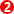and release your mouse-button.Blend-Curve: values at start-Point & end-Point. Start-Point•Angle = 90º, vertically upwards. The Blend-Curve is tangent with the vertical Line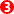. •Curvature = 0. (Radius-of-Curvature = ∞). It is a Straight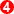•Curvature Rate = 0.  The Blend-Curve is a not changing at its start-Point.End-Point•Angle = –90º, vertically downwards. The Blend-Curve is tangent with the Arc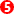. •Curvature  = 50 , (Radius of Curvature = 1/50 = 0.02m = 20mm).  Equal to the curvature of the Arc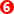. •Curvature Rate = 0.  The Curvature is not changing at its end-Point 2 .Note: Angle is counter-clockwise from X-axis of the Part to which you add the Blend-Curve. Open the Blend-Curve dialog-box STEP 4: If necessary, de-select Add Blend-Point STEP 5: Double-click the Blend-Curve to open the Blend-Curve dialog-box.Step 5 is Complete There are two separators in this dialog Start-Point parameters End-Point parameters Expand the Start-Point parameters separator: •Parameters equal our observations at the start-Point and end-Point, above.•Blend is selected for each parameter. Thus the parameters are Read-OnlyThese are the default settings. The Blend-Curve is continuous: •Angle•Curvature (Tangent) •Curvature Rate (Same Radius at the start-Point)Edit the Blend-Curve To the right the Curvature Rate in the Start Point and End-Point separators. STEP 6: Click the 'Blend' check-boxes, to de-select it STEP 7: Edit the Curvature RatesHere, I have changed the Curvature Rate values •Start-Point = 2400 •End-Point = -3000The units of Curvature Rate are 1/m2. Rather than calculate values for Curvature Rate, it is best to observe the shape of the Blend-Curve as you use the Spin-Box tool. What is the best value of the Blend-Curve? It is not possible to know unless you know the application of the Blend-Curve.This is the new 'shape' for the Blend-Curve.

#### Add a Motion Path FBThe Motion-Path FB is in the in the Kinematic-FB toolbar (R13.2: Kinematics-GB toolbar)STEP 1: Click Kinematic-FB toolbar > Add Motion-Path FB

STEP 2: Click the vertical Line

STEP 3: Click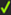in the Command-ManagerMotion-Path FBMotion-Point, at the start-Point of the sketch-element you selectDimension between the Motion-Point and the start-Point of the sketch-path - 0 (deg) because the Motion-Point is at the start-point.

The Dimension is not shown in the default case. Open the Motion-Path FB dialog-box > Point Params. tab > Point List... separator . You can show it as 'Displacement' or 'Phase'.

'Phase' normalizes the sketch-path between 0 and 360 degrees, with 0 at the start and 360 at the end of the sketch-path.

'Dimension' gives the literal distance from the start-Point to the Motion-Point.

 When sketch-element Points are 'merged', the Motion-Point will be at the start-Point of the sketch-Path and at the start-Point of the first sketch-element that you added. When sketch-elements Points are 'coincident', the Motion-Point will be at the start-Point of the sketch-element you select.

STEP 4: Add a Motion FB and a Linear-Motion FB

STEP 5: Edit the Motion FB to change the Output Data Type to Linear - in the Motion FB dialog-box

STEP 6: Connect the FBs in the usual way

Step 6 is CompleteSTEP 7: Cycle ( ALT+C )

You will see the Motion-Point move up the vertical line and then along the Blend-Curve.

It will stop, somewhere along the sketch-elements, then return to the start position.

What is the Motion?

The Motion that is in MotionDesigner and linked to the Motion FB.

Why only so far along the sketch-elements?

The Motion-Point moves the distance along the sketch-elements by the maximum Y-axis value in the Motion. In this case, 90mm.Cycle the Motion-Point between-: the ends of the sketch-elements

STEP 1: Double-click the Motion FB to open the Motion FB dialog-box

STEP 2: In the Output Data Type, click the Rotary radio button option

STEP 3: Click OKSTEP 4: Edit the Motion in MotionDesigner, to have a Rise of 360 units.

Why 360?

I find it easiest to think that the Motion-Point is a 'Crank'.

One complete rotation of the Crank = 360º.

One complete movement along the sketch-elements for the Motion-Point = 360 Rotary Units.The Motion-Point will move the complete length of the sketch-elements that are merged, when the:

Motion in MotionDesigner has a 'Rise' of 360 (units).

Output Data Type of the Motion FB is set to Rotary

See the Video, to the left, cycle the Motion-Point with the Motion in MotionDesigner.